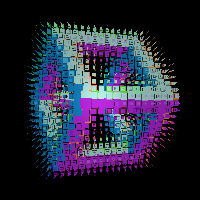# pointMapRender

Shortcuts:
• pmr
Keywords:
• point
• render
• volumetric
output
pointMapRender
RayTK
v0.36

Renderer that takes in a TOP of coordinates and evaluates the scene at each point.

The primary use for this is with TOP-based instancing for a Geometry COMP:

• Create a TOP with a coordinate value in each pixel. For example, this could be point cloud data, or converted points from a SOP/CHOP.
• Create your RayTK scene network, with an SDF or value/vector field.
• (Optionally) add a `sampledPointMat` to the scene to control how colors are applied to shapes.
• Create a `pointMapRender`, and connect the scene to the first input and the coordinate TOP to the TOP input.
• The TOP that comes out of the “Color” output will be a TOP with the same dimensions as the coordinate TOP, with a color value for each pixel, based on the scene.
• On a Geometry COMP, with instancing on, use the coordinate TOP for the instance positions, and use the colors TOP from the `pointMapRender` for the instance colors.

Other uses include:

• LED pixel mapping, where you use the physical location of each LED for the coordinates, and apply the resulting colors to each LED.
• GPU particles, where the simulation GLSL TOP produces coordinates from one buffer, which are run through the `pointMapRender`, and then feed the resulting values back into the simulation to control particle forces for each particle/pixel.

The “SDF or Value” output, when using an SDF scene, will output values where the RGB channels contain the distance to the surface. The alpha channel will be one for points that existed in the input map and zero for ones that did not. In other words the alpha channel will be the same as the alpha channel in the input point map.

## Parameters

• `Pixel Format`:
• `Use Input`:
• `8-bit fixed (RGBA)`:
• `sRGB 8-bit fixed (RGBA)`:
• `16-bit float (RGBA)`:
• `32-bit float (RGBA)`:
• `_separator_`:
• `10-bit RGB, 2-bit Alpha, fixed (RGBA)`:
• `16-bit fixed (RGBA)`:
• `11-bit float (RGB), Positive Values Only`:
• `16-bit float (RGB)`:
• `32-bit float (RGB)`:
• `8-bit fixed (Mono)`:
• `16-bit fixed (Mono)`:
• `16-bit float (Mono)`:
• `32-bit float (Mono)`:
• `8-bit fixed (RG)`:
• `16-bit fixed (RG)`:
• `16-bit float (RG)`:
• `32-bit float (RG)`:
• `8-bit fixed (A)`:
• `16-bit fixed (A)`:
• `16-bit float (A)`:
• `32-bit float (A)`:
• `8-bit fixed (Mono+Alpha)`:
• `16-bit fixed (Mono+Alpha)`:
• `16-bit float (Mono+Alpha)`:
• `32-bit float (Mono+Alpha)`:
• `Enable Normal Output`: Enable producing normal vectors. For each point, this will produce a vector pointing in the direction that the nearest surface point is facing. These values can be accessed using a `renderSelect` operator.
• `Enable Object Id Output`: Enable object ID output, which produces a TOP with values assigned with the `injectObjectId` operator for whichever shape each point is inside.
• `Time Reference Operator`:
• `Shader Builder Config`:
• `Coord Type`:
• `Auto`:
• `2D`:
• `3D`:
• `Output Type`:
• `2D Texture`:
• `2D Texture Array`:
• `3D Texture`:
• `Enable Debug Output`:
• `Enable Custom Output 1`:
• `Enable Custom Output 2`:
• `Enable Normal Smoothing`:
• `Normal Smoothing`:
• `Material Mode`:
• `Only Inside SDF`:
• `Only SDF Surface`:
• `Fill Everwhere`:
• `Enable Blending`:
• `Blending`:
• `Thickness`:
• `Customize Shader Config`:

## Inputs

• `SDF or Field`: (optional)
• Coordinate Types: `float` `vec2` `vec3` `vec4`
• Context Types: `Context`
• Return Types: `float` `vec4` `Sdf`
• `Camera`: (optional)
• Coordinate Types: `vec2`
• Context Types: `CameraContext`
• Return Types: `Ray`
• `Light`: (optional)
• Coordinate Types: `vec3`
• Context Types: `LightContext`
• Return Types: `Light`

## Variables

• `datapos`:
• `datauv`:
• `datares`: